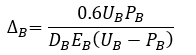top of page

# Fleming's method for pile settlement analysis in the PileLAT program

PileLAT predicts the pile settlement under compressive axial loading at the pile head. This unique feature is very useful. It enables the users to estimate the axial load settlement curve at the pile head when lateral force analysis is carried out without the need to set up a separate analysis file for pile settlement analysis.

The method proposed by Fleming (1992) is used to calculate the load settlement curve at the pile head. This method is based on the hyperbolic relationship assumptions for both shaft and base load-displacement responses. Elastic shortening of the pile is considered separately.

Pile settlement analysis can be carried out in PileLAT based on Fleming’s approach (Fleming 1992) published in Geotechnique. The pile settlement analysis inputs can be defined by the users with selecting "Pile Settlement Option" under "Options" menu as shown in the figure below.The input dialog for pile settlement analysis in PileLAT is shown in the figure below:The analysis requires the following inputs from the users:

• Dimensionless flexibility factor, Ms

• Effective length coefficient, Ke

• Soil stiffness at pile base, Eb

• Ultimate shaft resistance (fs) for each soil layer, and

• Ultimate end bearing resistance (fb) for each soil layer.

Based on the method proposed by Fleming (1992), the relationship between the pile displacement (Delta-S) and shaft load (Ps) is:where Ds is the pile diameter, Ps is the shaft load, Us is the ultimate total shaft resistance and Ms is the dimensionless flexibility factor ranging from 0.0001 to 0.004. Fleming (1992) suggested the value of approximately 0.0005 for soft rocks.

The hyperbolic relationship between the pile toe displacement (Delta-B) and pile base load (PB) is:where DB is the pile diameter at the base, PB is the pile toe load, UB is the ultimate pile toe bearing resistance force and EB is the Young’s Modulus of the material at the pile toe. The total load at the pile head, PT is calculated as:The elastic shortening (Delta-E) of the pile is determined with using the following equation to the load up to the ultimate shaft load, Us:For the greater load, the following equation is used to calculate the elastic shortening:where Ec is the Young’s modulus of the pile material, Lo is pile free length where no friction resistance from the soils, LF is length of the pile with friction load transfer and KE is effective length coefficient ranging from 0.4 to 0.5.

A typical output from PileLAT program for load settlement analysis based on Fleming's approach is shown in the figure below. Load-settlement curve is generated by the program for the specified axial loading at the pile head. The ultimate shaft resistance, ultimate end bearing resistance and ultimate axial pile capacity are calculated by the program and the results are shown on the load-settlement curve graph.The tabulated results of the load settlement curve at pile head can be viewed by the users with mouse right click on the graphical area of the load settlement curve and selecting the menu of “Show Table”. The tabulated results are shown in the table format as per the figure below.201 views